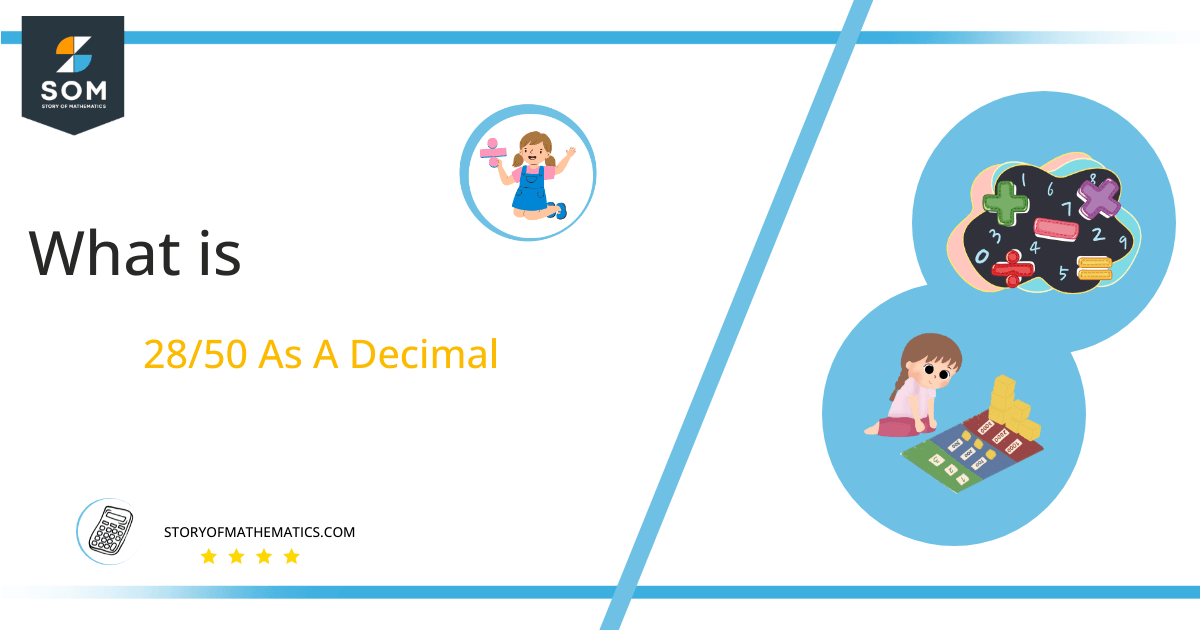# What Is 28/50 as a Decimal + Solution With Free Steps

The fraction 28/50 as a decimal is equal to 0.56.

A Fraction in arithmetic  is defined as a thing that depicts the number of parts contained by a specific size. Moreover, a complex fraction contains a fraction in the numerator or the denominator. In contrast, a Simple fraction contains both integers.

Here, we are more interested in the division types that result in a Decimal value, as this can be expressed as a Fraction. We see fractions as a way of showing two numbers having the operation of Division between them that result in a value that lies between two Integers.Now, we introduce the method used to solve said fraction to decimal conversion, called Long Division, which we will discuss in detail moving forward. So, let’s go through the Solution of fraction 28/50.

## Solution

First, we convert the fraction components, i.e., the numerator and the denominator, and transform them into the division constituents, i.e., the Dividend and the Divisor, respectively.

This can be done as follows:

Dividend = 28

Divisor = 50

Now, we introduce the most important quantity in our division process: the Quotient. The value represents the Solution to our division and can be expressed as having the following relationship with the Division constituents:

Quotient = Dividend $\div$ Divisor = 28 $\div$ 50

This is when we go through the Long Division solution to our problem.## 28/50 Long Division Method

We start solving a problem using the Long Division Method by first taking apart the division’s components and comparing them. As we have 28 and 50, we can see how 28 is Smaller than 50, and to solve this division, we require that 28 be Bigger than 50.

This is done by multiplying the dividend by 10 and checking whether it is bigger than the divisor or not. If so, we calculate the Multiple of the divisor closest to the dividend and subtract it from the Dividend. This produces the Remainder, which we then use as the dividend later.

Now, we begin solving for our dividend 28, which after getting multiplied by 10 becomes 280.

We take this 280 and divide it by 50; this can be done as follows:

280 $\div$ 50 $\approx$ 5

Where:

50 x 5 = 250

This will lead to the generation of a Remainder equal to 280– 250 = 30. Now this means we have to repeat the process by Converting the 30 into 300 and solving for that:

300 $\div$ 50 $\approx$ 6

Where:

50 x 6 = 300

This, therefore, produces another Remainder which is equal to 300 – 300 = 0.Images/mathematical drawings are created with GeoGebra.